# Polygram (geometry)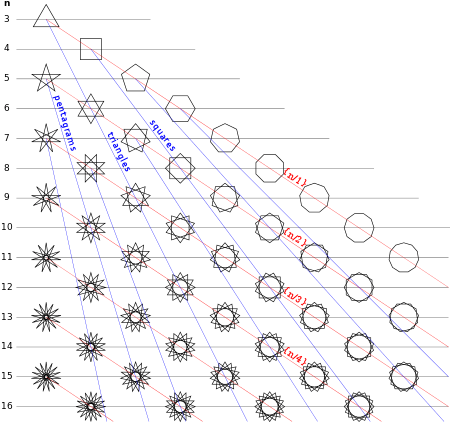Regular polygrams {n/d}, with red lines showing constant d, and blue lines showing compound sequences k{n/d}

In geometry, a generalized polygon can be called a polygram, and named specifically by its number of sides, so a regular pentagram, {5/2}, has 5 sides, and the regular hexagram, {6/2} or 2{3} has 6 sides divided into two triangles.

A regular polygram {p/q} can either be in a set of regular polygons (for gcd(p,q)=1, q>1) or in a set of regular polygon compounds (if gcd(p,q)>1).

## Etymology

The polygram names combine a numeral prefix, such as penta-, with the Greek suffix -gram (in this case generating the word pentagram). The prefix is normally a Greek cardinal, but synonyms using other prefixes exist. The -gram suffix derives from γραμμῆς (grammos) meaning a line.

## Generalized regular polygons

A regular polygram, as a general regular polygon, is denoted by its Schläfli symbol {p/q}, where p and q are relatively prime (they share no factors) and q  2. For integers p and q, it can be considered as being constructed by connecting every qth point out of p points regularly spaced in a circular placement.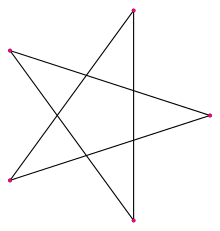{5/2}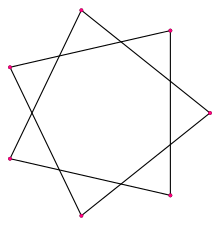{7/2}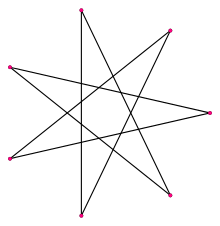{7/3}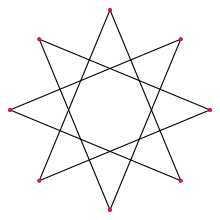{8/3}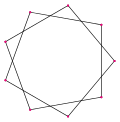{9/2}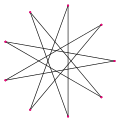{9/4}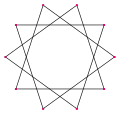{10/3}...

## Regular compound polygons

In other cases where n and m have a common factor, a polygram is interpreted as a lower polygon, {n/k,m/k}, with k = gcd(n,m), and rotated copies are combined as a compound polygon. These figures are called regular compound polygons.

Some regular polygon compounds
Triangles... Squares... Pentagons... Pentagrams...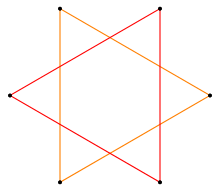{6/2}=2{3}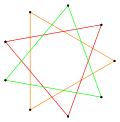{9/3}=3{3}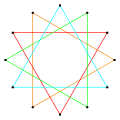{12/4}=4{3}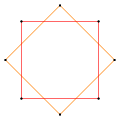{8/2}=2{4}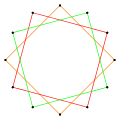{12/3}=3{4}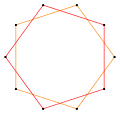{10/2}=2{5}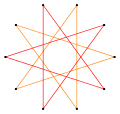{10/4}=2{5/2}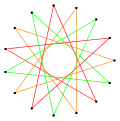{15/6}=3{5/2}